### Solution of Hydrogen Radial Equation *

The differential equation we wish to solve is.First we change to a dimensionless variable,giving the differential equationwhere the constantNext we look at the equation for large.This can be solved by, so we explicitly include this.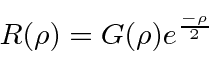We should also pick of the smallbehavior.Assuming, we get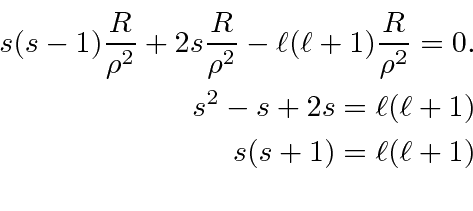So eitheror. The second is not well normalizable. We write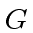as a sum.The differential equation forisWe plug the sum into the differential equation.Now we shift the sum so that each term contains.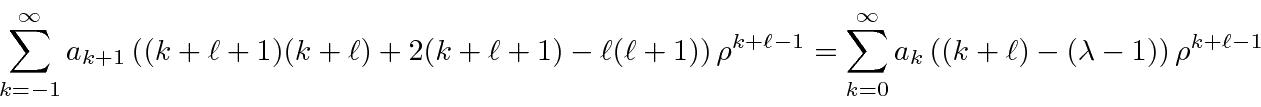The coefficient of each power ofmust be zero, so we can derive the recursion relation for the constants.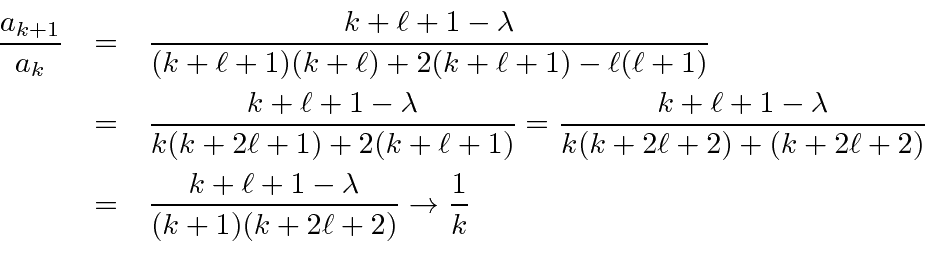This is then the power series forunless it somehow terminates. We can terminate the series if for some value of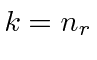,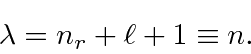The number of nodes inwill be. We will callthe principal quantum number, since the energy will depend only on.

Plugging in for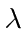we get the energy eigenvalues.The solutions areThe recursion relation isWe can rewrite, substituting the energy eigenvalue.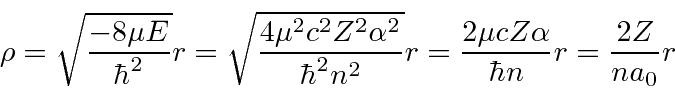Jim Branson 2013-04-22